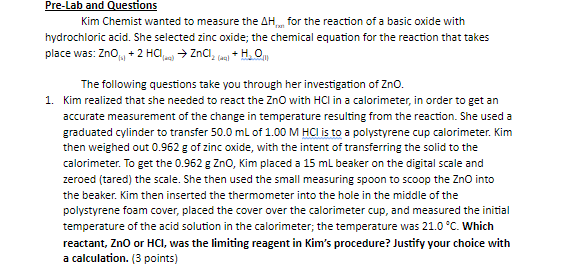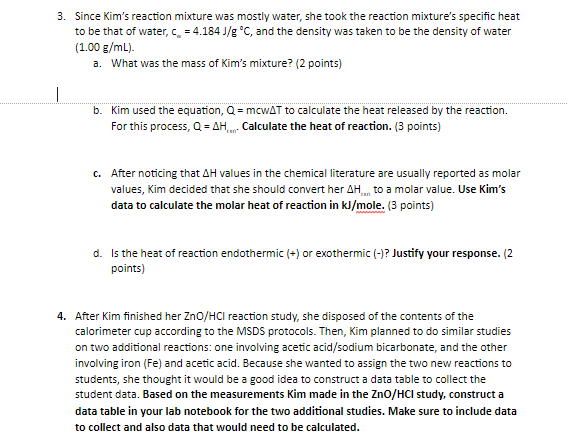# Question Solved1 AnswerFinal Temp. = 25.4 degrees Celsius Pre-Lab and Questions Kim Chemist wanted to measure the AH, for the reaction of a basic oxide with hydrochloric acid. She selected zinc oxide; the chemical equation for the reaction that takes place was: ZnO, + 2 HCl → ZnCla+HO The following questions take you through her investigation of Zno. 1. Kim realized that she needed to react the ZnO with HCl in a calorimeter, in order to get an accurate measurement of the change in temperature resulting from the reaction. She used a graduated cylinder to transfer 50.0 mL of 1.00 M HCl is to a polystyrene cup calorimeter. Kim then weighed out 0.962 g of zinc oxide, with the intent of transferring the solid to the calorimeter. To get the 0.962 g Zno, Kim placed a 15 ml beaker on the digital scale and zeroed (tared) the scale. She then used the small measuring spoon to scoop the ZnO into the beaker. Kim then inserted the thermometer into the hole in the middle of the polystyrene foam cover, placed the cover over the calorimeter cup, and measured the initial temperature of the acid solution in the calorimeter; the temperature was 21.0 °C. Which reactant, ZnO or HCI, was the limiting reagent in Kim's procedure? Justify your choice with a calculation. (3 points) 3. Since Kim's reaction mixture was mostly water, she took the reaction mixture's specific heat to be that of water, c = 4.184 J/g °C, and the density was taken to be the density of water (1.00 g/mL). a. What was the mass of Kim's mixture? (2 points) b. Kim used the equation, Q = mcWAT to calculate the heat released by the reaction. For this process, Q = AH... Calculate the heat of reaction. (3 points) C. After noticing that AH values in the chemical literature are usually reported as molar values, Kim decided that she should convert her AH...to a molar value. Use Kim's data to calculate the molar heat of reaction in kJ/mole. (3 points) d. Is the heat of reaction endothermic (+) or exothermic (-)? Justify your response. (2 points) 4. After Kim finished her ZnO/HCl reaction study, she disposed of the contents of the calorimeter cup according to the MSDS protocols. Then, Kim planned to do similar studies on two additional reactions: one involving acetic acid/sodium bicarbonate, and the other involving iron (Fe) and acetic acid. Because she wanted to assign the two new reactions to students, she thought it would be a good idea to construct a data table to collect the student data. Based on the measurements Kim made in the ZnO/HCI study, construct a data table in your lab notebook for the two additional studies. Make sure to include data to collect and also data that would need to be calculated.Final Temp. = 25.4 degrees Celsius
Transcribed Image Text: Pre-Lab and Questions Kim Chemist wanted to measure the AH, for the reaction of a basic oxide with hydrochloric acid. She selected zinc oxide; the chemical equation for the reaction that takes place was: ZnO, + 2 HCl → ZnCla+HO The following questions take you through her investigation of Zno. 1. Kim realized that she needed to react the ZnO with HCl in a calorimeter, in order to get an accurate measurement of the change in temperature resulting from the reaction. She used a graduated cylinder to transfer 50.0 mL of 1.00 M HCl is to a polystyrene cup calorimeter. Kim then weighed out 0.962 g of zinc oxide, with the intent of transferring the solid to the calorimeter. To get the 0.962 g Zno, Kim placed a 15 ml beaker on the digital scale and zeroed (tared) the scale. She then used the small measuring spoon to scoop the ZnO into the beaker. Kim then inserted the thermometer into the hole in the middle of the polystyrene foam cover, placed the cover over the calorimeter cup, and measured the initial temperature of the acid solution in the calorimeter; the temperature was 21.0 °C. Which reactant, ZnO or HCI, was the limiting reagent in Kim's procedure? Justify your choice with a calculation. (3 points) 3. Since Kim's reaction mixture was mostly water, she took the reaction mixture's specific heat to be that of water, c = 4.184 J/g °C, and the density was taken to be the density of water (1.00 g/mL). a. What was the mass of Kim's mixture? (2 points) b. Kim used the equation, Q = mcWAT to calculate the heat released by the reaction. For this process, Q = AH... Calculate the heat of reaction. (3 points) C. After noticing that AH values in the chemical literature are usually reported as molar values, Kim decided that she should convert her AH...to a molar value. Use Kim's data to calculate the molar heat of reaction in kJ/mole. (3 points) d. Is the heat of reaction endothermic (+) or exothermic (-)? Justify your response. (2 points) 4. After Kim finished her ZnO/HCl reaction study, she disposed of the contents of the calorimeter cup according to the MSDS protocols. Then, Kim planned to do similar studies on two additional reactions: one involving acetic acid/sodium bicarbonate, and the other involving iron (Fe) and acetic acid. Because she wanted to assign the two new reactions to students, she thought it would be a good idea to construct a data table to collect the student data. Based on the measurements Kim made in the ZnO/HCI study, construct a data table in your lab notebook for the two additional studies. Make sure to include data to collect and also data that would need to be calculated.
More
Transcribed Image Text: Pre-Lab and Questions Kim Chemist wanted to measure the AH, for the reaction of a basic oxide with hydrochloric acid. She selected zinc oxide; the chemical equation for the reaction that takes place was: ZnO, + 2 HCl → ZnCla+HO The following questions take you through her investigation of Zno. 1. Kim realized that she needed to react the ZnO with HCl in a calorimeter, in order to get an accurate measurement of the change in temperature resulting from the reaction. She used a graduated cylinder to transfer 50.0 mL of 1.00 M HCl is to a polystyrene cup calorimeter. Kim then weighed out 0.962 g of zinc oxide, with the intent of transferring the solid to the calorimeter. To get the 0.962 g Zno, Kim placed a 15 ml beaker on the digital scale and zeroed (tared) the scale. She then used the small measuring spoon to scoop the ZnO into the beaker. Kim then inserted the thermometer into the hole in the middle of the polystyrene foam cover, placed the cover over the calorimeter cup, and measured the initial temperature of the acid solution in the calorimeter; the temperature was 21.0 °C. Which reactant, ZnO or HCI, was the limiting reagent in Kim's procedure? Justify your choice with a calculation. (3 points) 3. Since Kim's reaction mixture was mostly water, she took the reaction mixture's specific heat to be that of water, c = 4.184 J/g °C, and the density was taken to be the density of water (1.00 g/mL). a. What was the mass of Kim's mixture? (2 points) b. Kim used the equation, Q = mcWAT to calculate the heat released by the reaction. For this process, Q = AH... Calculate the heat of reaction. (3 points) C. After noticing that AH values in the chemical literature are usually reported as molar values, Kim decided that she should convert her AH...to a molar value. Use Kim's data to calculate the molar heat of reaction in kJ/mole. (3 points) d. Is the heat of reaction endothermic (+) or exothermic (-)? Justify your response. (2 points) 4. After Kim finished her ZnO/HCl reaction study, she disposed of the contents of the calorimeter cup according to the MSDS protocols. Then, Kim planned to do similar studies on two additional reactions: one involving acetic acid/sodium bicarbonate, and the other involving iron (Fe) and acetic acid. Because she wanted to assign the two new reactions to students, she thought it would be a good idea to construct a data table to collect the student data. Based on the measurements Kim made in the ZnO/HCI study, construct a data table in your lab notebook for the two additional studies. Make sure to include data to collect and also data that would need to be calculated.